# Roman numeral facts for kids

Kids Encyclopedia Facts

A Roman numeral is the name for a number when it is written in the way the Romans used to write numbers. Roman numerals are not used very often today in the west. They are used to write the names of kings and queens, or popes. For example: Queen Elizabeth II. They may be used to write the year a book or movie was made.Did you know the Romans had no 0 in Roman numerals?

## The base

 Numeral Value I V X L C D M ↁ ↂ 1 5 10 50 100 500 1,000 5,000 10,000

In general, the system is additive, which means that the numbers are added.

As an example 'VI' represents the number 6, 'XIII' is 13.

## Subtraction rule

There is a simple rule, which generally avoids that more than three of the same symbols are added. It says that the lower value symbol which is directly before the higher one, is subtracted, and not added. This rule was used in Roman times, but not at all times. It is used since about the Middle Ages. This means that rather than writing 'IIII', it is preferable to write 'IV', for the number 4. Also note that the subtraction rule is only valid for the symbol which comes right beforehand in the sequence. This means that 99 is written XCIX, and not IC.

## Fractions

The Romans also used fractions. The most common base for fractions was 1/12, which in Latin is called uncia. The word uncia transformed to the modern English word ounce. There are special names for fractions where the numerator is one less than the denominator, an example of this is ''Dodrans'' (de quadrans,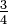$\tfrac{3}{4}$).

 Fraction Name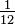$\tfrac{1}{12}$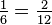$\tfrac{1}{6}=\tfrac{2}{12}$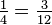$\tfrac{1}{4}=\tfrac{3}{12}$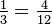$\tfrac{1}{3}=\tfrac{4}{12}$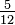$\tfrac{5}{12}$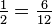$\tfrac{1}{2}=\tfrac{6}{12}$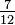$\tfrac{7}{12}$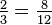$\tfrac{2}{3}=\tfrac{8}{12}$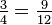$\tfrac{3}{4}=\tfrac{9}{12}$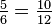$\tfrac{5}{6}=\tfrac{10}{12}$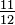$\tfrac{11}{12}$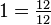$1=\tfrac{12}{12}$ Uncia (Twelfth) Sextans (Sixth) Quadrans (Fourth) Triens (Third) Quincunx (quinque unciae) Semis (Half) Septunx (septem unciae) Bes, Bessis (bis triens; dues partes Asis) Dodrans (de quadrans) Dextans (de sextans) Deunx (de uncia) As

## Using roman numerals to write years

It is very easy to write a number as a Roman numeral. Simply substract the largest possible Roman numeral, as many times as possible from the number. This system will result in a valid Roman numeral, but will not take the subtraction rule into account.

 1 × 1000 + 1 × 500 + 4 × 100 + 1 × 50 + 3 × 10 + 4 × 1 = 1984 M + D + CCCC + L + XXX + IIII = MDCCCCLXXXIIII

Getting the number from the numeral is equally simple, by adding the values of the symbols.

In general, the values for 5, 50, 500,.. are not subtracted. The same number, with using the subtraction rule:

 1 × 1000 + (−1 × 100 + 1 × 1000) + 1 × 50 + 3 × 10 + (−1 × 1 + 1 × 5) = 1984 M + CM + L + XXX + IV = MCMLXXXIV

There are two exceptions to the subtraction rule:

• The value to be subtracted occurs twice, and is subtracted twice. IIX, instead of VIII for 8
• I and X are not only used before the next higher value (V, and L) but also higher values: IL instead of XLIX for 49, IC instead of XCIX for 99, XM instead of CMXC for 990

Both of these exceptions can be combined: IIL instead of XLVIII for 48, IIC instead of XCVIII for 98.

## Notation

The system that is in use today is: whenever the same symbol is written four times, it is replaced by subtracting it from the next higher number (5,50,50,500). That way, IV is written instead of IIII (4), XL instead of XXXX (40), etc.

Usually only one number is subtracted, not two. So 8 is always VIII and never IIX

Especially on clocks and watches, IIII can sometimes still be found. This is done partly because the IIII for the 4 o'clock position aesthetically balances the VIII for the 8 o'clock position. Also, some use it as a metaphor that IIII was commonplace in an period of time earlier than the idea of subtraction - IX - was introduced into writing Roman numerals. (4:00 comes earlier in the day than 9:00.)

Proper form is to subtract only a value with the next lower power of 10. Thus, 900 is written CM, but 990 would not be XM - properly, it is CM for the 900 portion and XC for the 90 portion, or CMXC. Similarly, 999 would not be IM but rather CMXCIX - CM for the 900 portion, XC for the 90 portion, and IX for the 9 portion. Only values with 1's are ever used to subtract; 45 is properly XLV, not VL.

Notations in Roman numerals for numbers higher than 3,001 are rarely seen. One system uses V and X with bars over them to signify 5,000 and 10,000, respectively. So in the lists above, though 5000 etc may appear as V when writing it add a bar on top.

Unicode has a code block called Number Forms, which also contains representations of Roman numerals, at the positions U+2160 to U+2188.

## What they are used for

• In the Baltics and Russia, the days of the week, are often written as Roman numbers, I being Monday.
• When writing dates by hand, the month is sometimes written as a Roman numeral, especially for dates written in day-month-year sequence. Example: 26.XI.2014 = November 26, 2014.
• When movies or books are published, the year of publication or year of copyright may be done as a Roman numeral.
• When people write about Monarchs or Popes, Patriarchs, or other leading figures, they are sometimes counted with Roman numbers, e.g. Queen Elizabeth II (of England), Pope John Paul II, Pope Benedict XVI, Patriarch Alexius II (of the Russian-Orthodox church)
• In France, the trimesters are sometimes counted with Roman numerals.
• In Poland, roman numerals are used to show the month in dates and as a short method of writing ordinals (i.e. VI to be 6th)

## Images for kidsRoman numeral Facts for Kids. Kiddle Encyclopedia.